## 第二周 自然语言处理与词嵌入（Natural Language Processing and Word Embeddings）

### 2.1 词汇表征（Word Representation）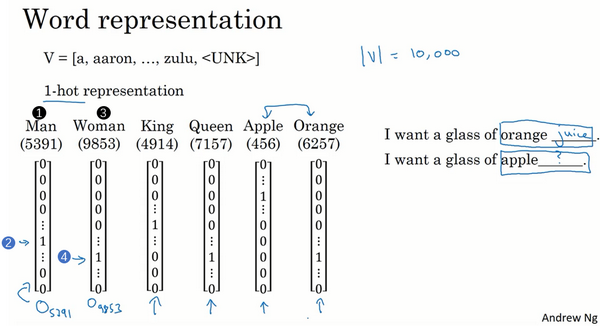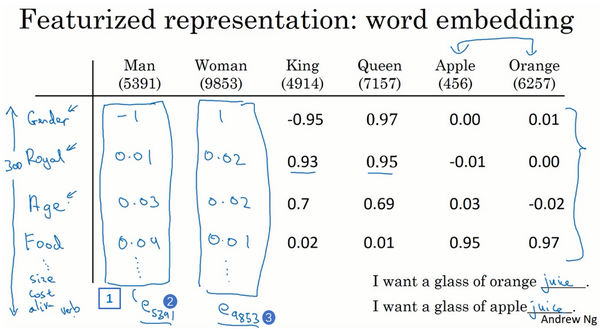### 2.2 使用词嵌入（Using Word Embeddings）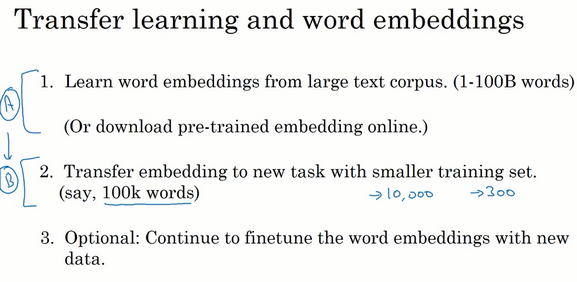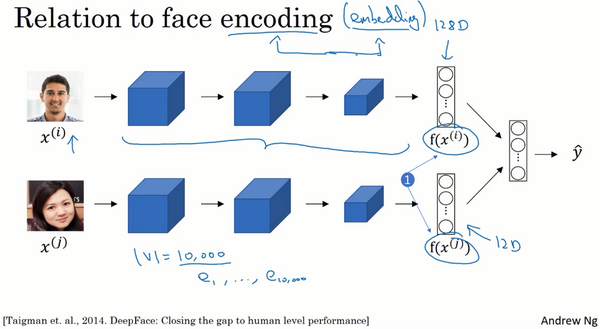### 2.3 词嵌入的特性（Properties of Word Embeddings）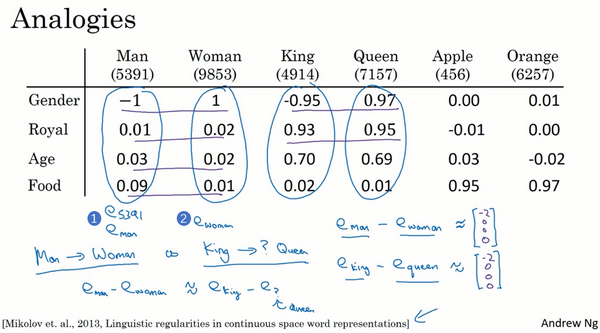Mikolov T, Yih W T, Zweig G. Linguistic regularities in continuous space word representations[J]. In HLT-NAACL, 2013.$Find\ word\ w:argmax \ Sim(e_{w},e_{\text{king}} - e_{\text{man}} + e_{\text{woman}})$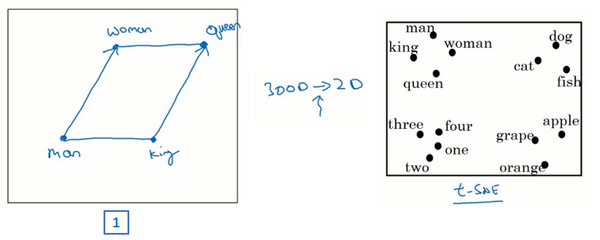### 2.4 嵌入矩阵（Embedding Matrix）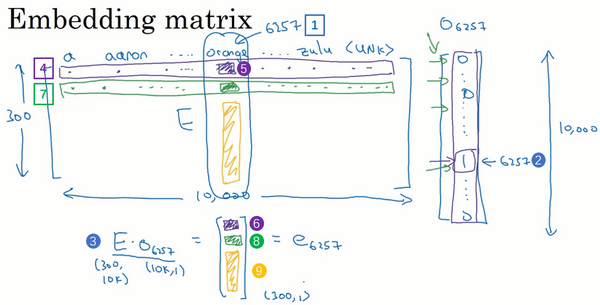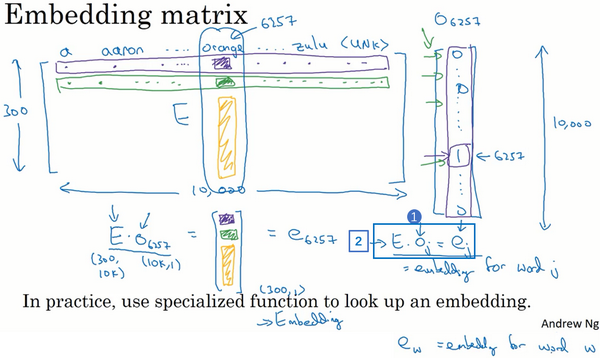### 2.5 学习词嵌入（Learning Word Embeddings）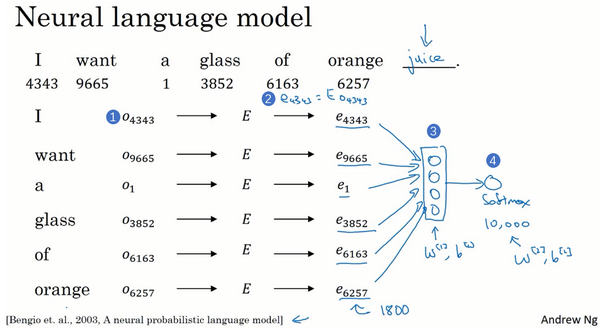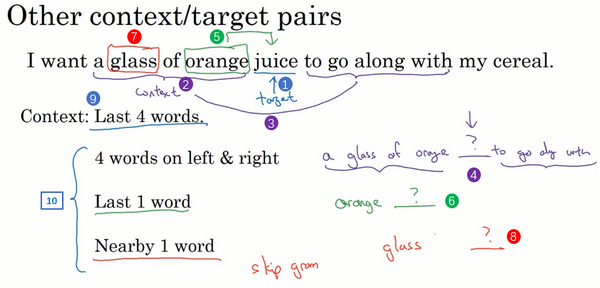### 2.6 Word2Vec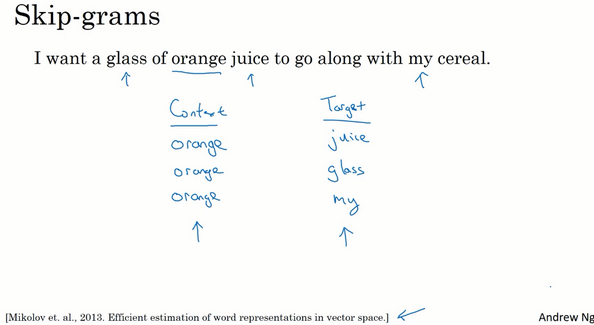Mikolov T, Chen K, Corrado G, et al. Efficient Estimation of Word Representations in Vector Space[J]. Computer Science, 2013.$Softmax:p\left( t \middle| c \right) = \frac{e^{\theta_{t}^{T}e_{c}}}{\sum_{j = 1}^{10,000}e^{\theta_{j}^{T}e_{c}}}$

$L\left( \hat y,y \right) = - \sum_{i = 1}^{10,000}{y_{i}\log \hat y_{i}}$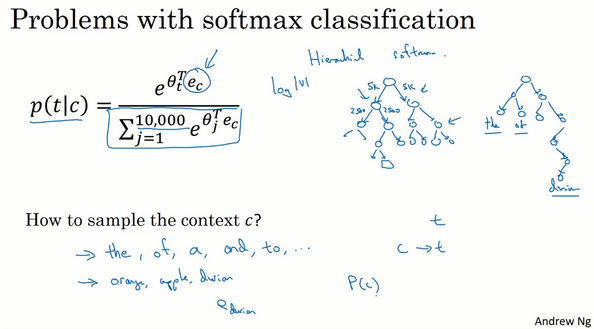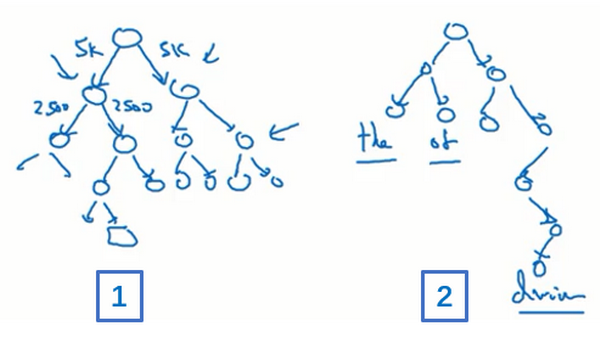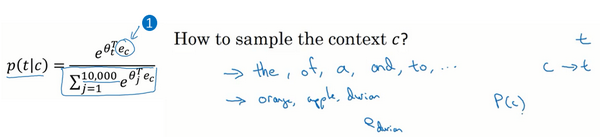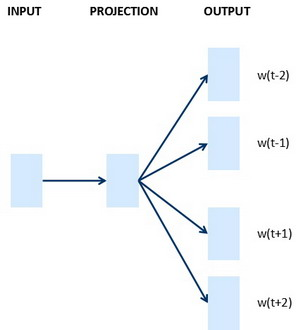### 2.7 负采样（Negative Sampling）

Mikolov T, Sutskever I, Chen K, et al. Distributed Representations of Words and Phrases and their Compositionality[J]. 2013, 26:3111-3119.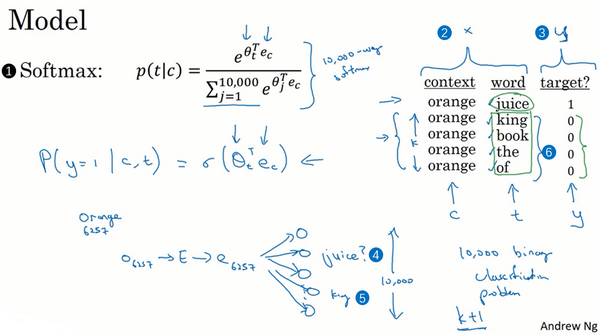$P\left( y = 1 \middle| c,t \right) = \sigma(\theta_{t}^{T}e_{c})$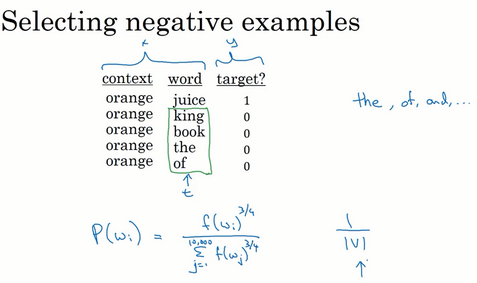$P\left( w_{i} \right) = \frac{f\left( w_{i} \right)^{\frac{3}{4}}}{\sum_{j = 1}^{10,000}{f\left( w_{j} \right)^{\frac{3}{4}}}}$

Skip-Gram模型就介绍到这里，在下个视频中，我会跟你分享另一个版本的词嵌入学习算法GloVe，而且这可能比你之前看到的都要简单。

### 2.8 GloVe 词向量（GloVe Word Vectors）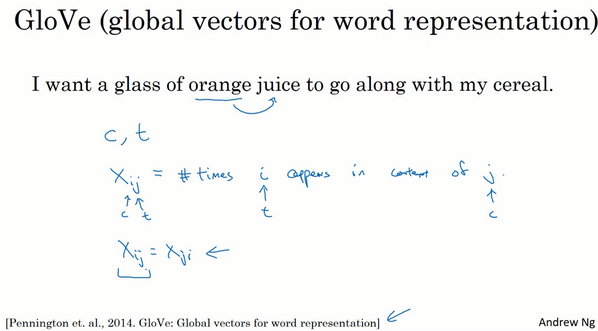Glove算法是由Jeffrey PenningtonRichard SocherChris Manning发明的。

(Pennington J, Socher R, Manning C. Glove: Global Vectors for Word Representation[C]// Conference on Empirical Methods in Natural Language Processing. 2014:1532-1543.)

GloVe代表用词表示的全局变量（global vectors for word representation）。在此之前，我们曾通过挑选语料库中位置相近的两个词，列举出词对，即上下文和目标词，GloVe算法做的就是使其关系开始明确化。假定$X_{{ij}}$是单词$i$在单词$j$上下文中出现的次数，那么这里$i$$j$就和$t$$c$的功能一样，所以你可以认为$X_{{ij}}$等同于$X_{{tc}}$。你也可以遍历你的训练集，然后数出单词$i$在不同单词$j$上下文中出现的个数，单词$t$在不同单词$c$的上下文中共出现多少次。根据上下文和目标词的定义，你大概会得出$X_{{ij}}$等于$X_{ji}$这个结论。事实上，如果你将上下文和目标词的范围定义为出现于左右各10词以内的话，那么就会有一种对称关系。如果你对上下文的选择是，上下文总是目标词前一个单词的话，那么$X_{{ij}}$$X_{ji}$就不会像这样对称了。不过对于GloVe算法，我们可以定义上下文和目标词为任意两个位置相近的单词，假设是左右各10词的距离，那么$X_{{ij}}$就是一个能够获取单词$i$和单词$j$出现位置相近时或是彼此接近的频率的计数器。

GloVe模型做的就是进行优化，我们将他们之间的差距进行最小化处理：

$\text{mini}\text{mize}\sum_{i = 1}^{10,000}{\sum_{j = 1}^{10,000}{f\left( X_{{ij}} \right)\left( \theta_{i}^{T}e_{j} + b_{i} + b_{j}^{'} - logX_{{ij}} \right)^{2}}}$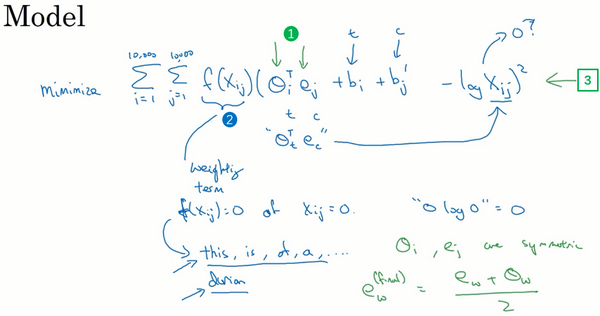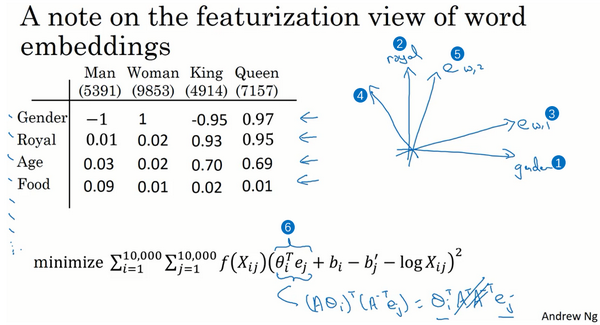$\left( A\theta_{i} \right)^{T}\left( A^{- T}e_{j} \right) = \theta_{i}^{T}A^{T}A^{- T}e_{j} = \theta_{i}^{T}e_{j}$

### 2.9 情感分类（Sentiment Classification）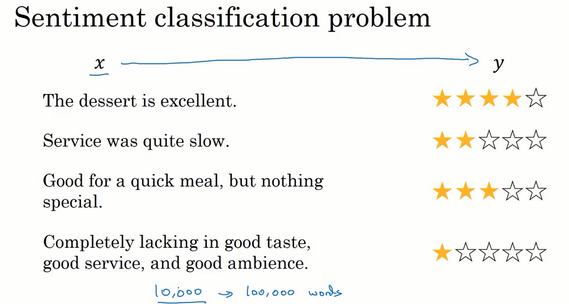"Service was quite slow"（服务太慢），两星评价；

"Good for a quick meal but nothing special"（适合吃快餐但没什么亮点），三星评价；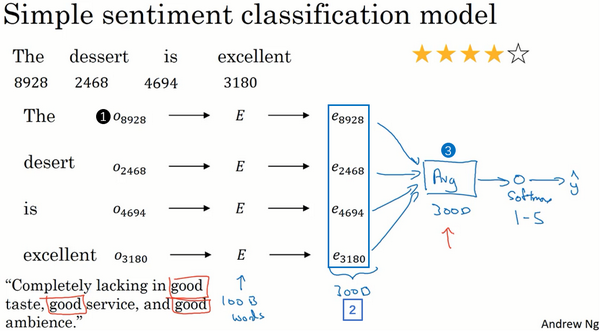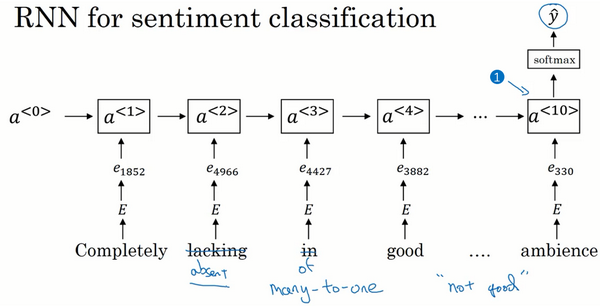### 2.10 词嵌入除偏（Debiasing Word Embeddings）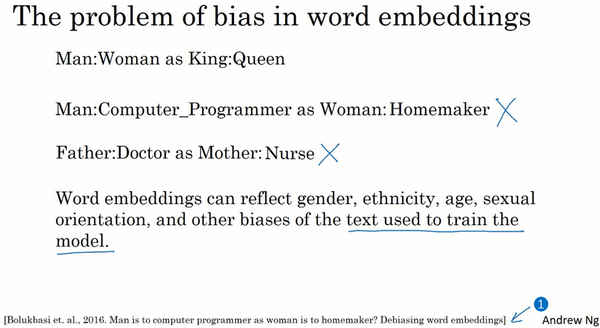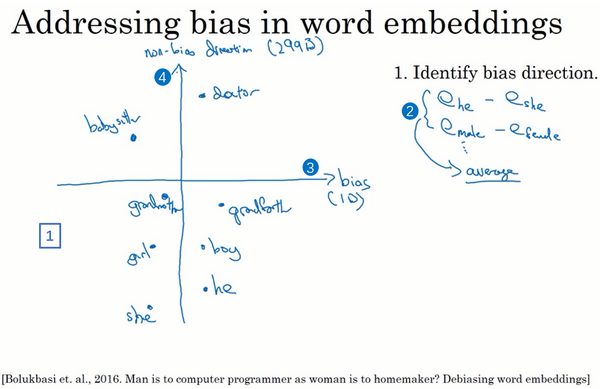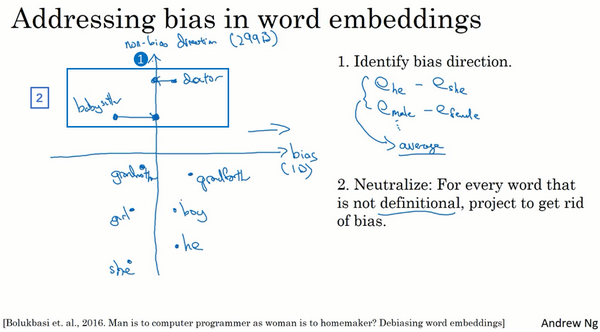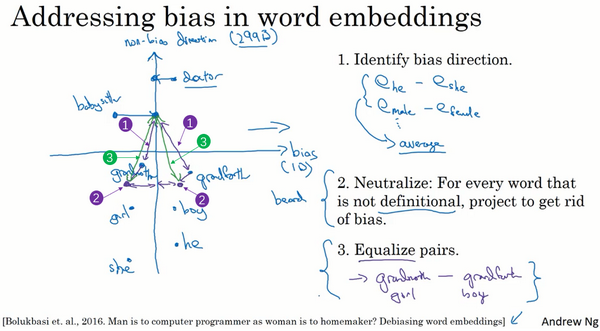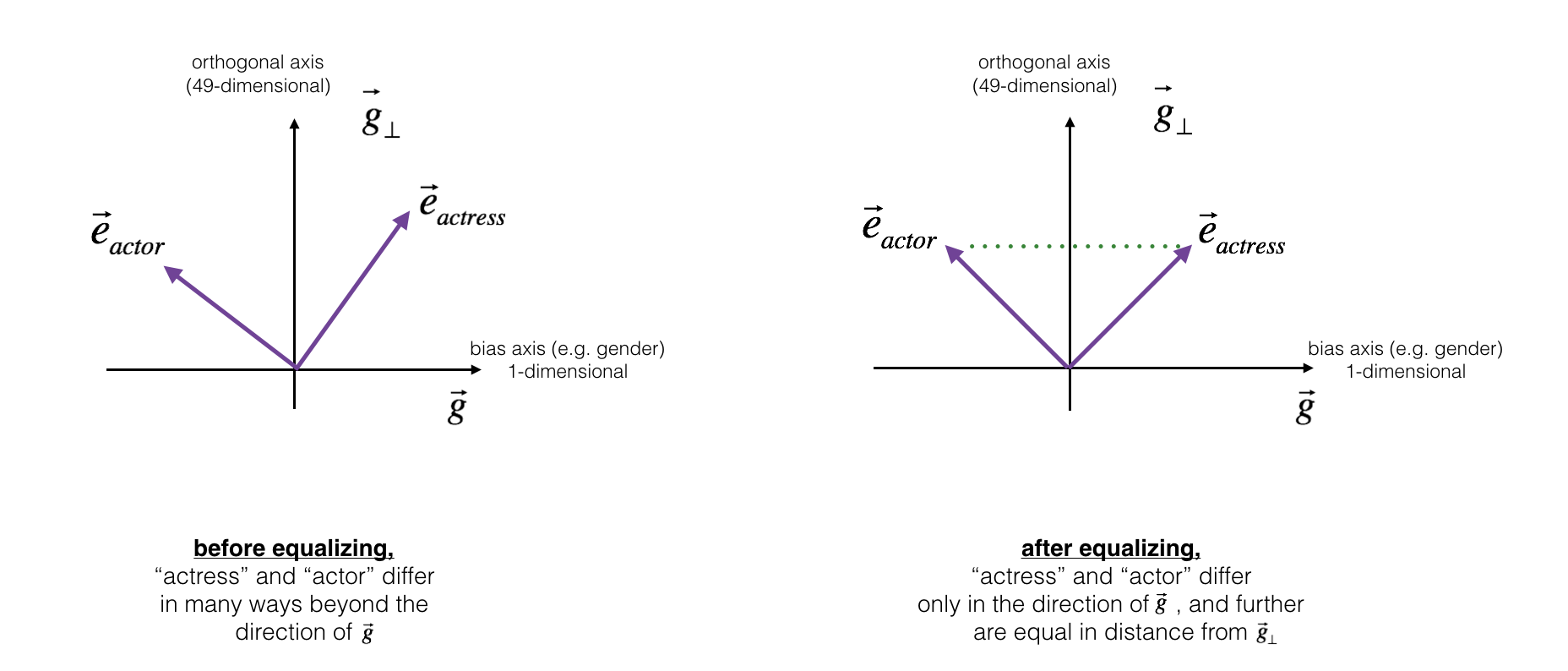$\mu = \frac{e_{w1} + e_{w2}}{2}\tag{1}$

$\mu_{B} = \frac {\mu * \text{bias_axis}}{||\text{bias_axis}||_2} + ||\text{bias_axis}||_2 *\text{bias_axis}\tag{2}$

$\mu_{\perp} = \mu - \mu_{B} \tag{3}$

$e_{w1B} = \sqrt{ |{1 - ||\mu_{\perp} ||^2_2} |} * \frac{(e_{{w1}} - \mu_{\perp}) - \mu_B} {|(e_{w1} - \mu_{\perp}) - \mu_B)|} \tag{4}$

$e_{w2B} = \sqrt{ |{1 - ||\mu_{\perp} ||^2_2} |} * \frac{(e_{\text{w2}} - \mu_{\perp}) - \mu_B} {|(e_{w2} - \mu_{\perp}) - \mu_B)|} \tag{5}$

$e_1 = e_{w1B} + \mu_{\perp} \tag{6}$ $e_2 = e_{w2B} + \mu_{\perp} \tag{7}$